Next: Analysis III - Molecular Up: Measurement of the Ramsauer-Townsend Previous: Monte Carlo parameter determination

## The Ramsauer-Townsend minimum energy

With these Monte Carlo parameters fixed, we can now compare the time of flight spectrum of the emitted muonic tritium with Monte Carlo simulations to test theoretical cross sections based on few-body theory. We shall focus on the measurement of the position of the Ramsauer-Townsend minimum ER, since this is important both as a source of the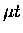beam and as a test of the quantum three-body calculations.

The Nuclear Atlas cross sections [16,17] for the muonic processes were used as a nominal input to the Monte Carlo. Molecular and condensed matter effects  [167,170,216] are expected to play negligible roles in the transport properties of muonic atoms at the energies above a few eV. Iterative calculations were performed by multiplying the energy scale of theelastic scattering cross section by a constant factor, thus shifting the RT minimum as . The resulting simulated time spectrum is fitted to the experimental data with one free parameter (relative normalization), and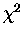was calculated for each value of. For both the data and the MC, a longitudinal spatial cut of z=[-10,10] mm was applied to select the vacuum region.

Figure 7.4 shows an example of such a fit (top) and its residuals (bottom). Plotted with error bars is thetime spectrum from the standard emission target(1000 T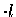H2 with 0.1% T2 ) from which a background runof pure 1000 TH2, normalized to GMU, was subtracted, while the histogram shows a simulated time spectrum assuming the energy scaling factor of , which turned out to give the best DOF of 1.06 for fit interval t=[0,6]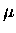s (region A) with DOF =59. Plotted in the bottom of Fig. 7.4 is the fit residual, i.e. the difference between the Monte Carlo and the data, normalized to one standard deviation.

Illustrated in Fig. 7.5 is the global trend of totalversus the energy scaling factor, while Fig. 7.6 shows the details near the minimum. The horizontal axis is plotted against the inverse of square root ofto reflect our sensitivity to the time of flight, rather than the energy.

Dependences of the various parameters were investigated in detail for the potential systematic effects. Figs. 7.5 and  7.6 show some examples of such investigations including:

1.
Muon transfer rate from protons to tritons, , as input to the simulation. Our standard input uses an experimental rate measured by our collaboration, s-1  for this process, but fits using the theoretical energy-dependent value of from the Nuclear Atlas cross section  were also tried.
2.
Time interval of the fit region. Region A: t=[0,6]s, Region B: t=[0.1,3]s (see Fig. 7.4). If our fit model is correct, the fit results should be independent of fit region except to add a constant value in total, which was confirmed in Figs. 7.57.6.
3.
MWPC resolution parameter . Our nominal value 1.3 mm was varied from 1.0 mm to 1.5 mm (only some selections are shown in Fig. 7.6.)
4.
Beam width parameters characterizing a flat top Gaussian distribution in the XY plane. 1010 mm (nominal) and 1515 mm were plotted.
5.
Muon beam stopping distribution in the z direction of the emission layer.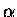: Gaussian with the peak at the surface of the layer with the standard deviation width of half the layer thickness.
: Gaussian with the peak at the centre of the layer with the standard deviation width of 0.4 times layer thickness.
No symbol: uniform Z stopping distribution.

For the nominal values of parameters, we obtained the best fit with ( in the figures). The value ofshifted between 1.05 to 1.15 depending on the parameters (see Fig 7.6). In addition, we estimate that the uncertainty in the drift distance scale can give rise to a shift inof order . With the two major systematic errors (due to the parameters (1)-(5) and the distance scale) added in quadrature, and the statistical errors much smaller, as can be observed in Fig. 7.6, our measurement indicates a scaling factor of , i.e., the Ramsauer-Townsend minimum energy of eV (c.f. the theortecial minimum, ERth = 12.4 eV). The results of a similar analysis for a measurement using an emission target with a tritium concentration of 0.3%were consistent with .

In conclusion, we have reported in this chapter, (1) the first observation ofin vacuum, (2) the first quantitative spectroscopic evidence of the Ramsauer-Townsend effect in an exotic system, confirming the theoretical RT minimum energy in theelastic scattering at the 10% level, an accuracy sufficent for our goal of molecular formation rate measurements.Next: Analysis III - Molecular Up: Measurement of the Ramsauer-Townsend Previous: Monte Carlo parameter determination